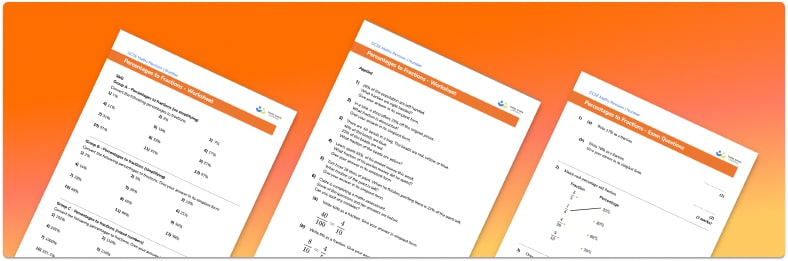# Percentages to Fractions Worksheet• Section 1 of the percentages to fractions worksheet contains 36 skills-based percentages to fractions questions, in 3 groups to support differentiation
• Section 2 contains 6 applied percentages to fractions questions with a mix of word problems and deeper problem solving questions
• Section 3 contains 4 foundation and higher level GCSE exam style practice questions including word problems
• Answers and a mark scheme for all questions are provided
• Questions follow variation theory with plenty of opportunities for students to work independently at their own level
• All questions are created by fully qualified expert secondary maths teachers
• Suitable for GCSE maths revision for AQA, OCR and Edexcel exam boards

• This field is for validation purposes and should be left unchanged.

You can unsubscribe at any time (each email we send will contain an easy way to unsubscribe). To find out more about how we use your data, see our privacy policy.

### Percentages to fractions at a glance

Percent means out of 100 and so to convert a percentage to a fraction, we put the percentage as the numerator and 100 as the denominator of the fraction. For example, 47% is equal to 47 divided by 100. 47% is the same as 47 hundredths.

Once we have written the fraction, we can simplify it until it is in its simplest form. Percentages greater than 100% can still be written as fractions – this will lead to an improper fraction which could then be written as a mixed number. If the percentage is a decimal, the numerator and denominator of the fraction will need to be multiplied to make the numerator a whole number.

Looking forward, students can then progress to additional fractions, decimals and percentages worksheets and number worksheets, for example a multiplying and dividing decimals worksheetFor more teaching and learning support on Number our GCSE maths lessons provide step by step support for all GCSE maths concepts.

## Do you have KS4 students who need more focused attention to succeed at GCSE?There will be students in your class who require individual attention to help them succeed in their maths GCSEs. In a class of 30, it’s not always easy to provide.

Help your students feel confident with exam-style questions and the strategies they’ll need to answer them correctly with our dedicated GCSE maths revision programme.

Lessons are selected to provide support where each student needs it most, and specially-trained GCSE maths tutors adapt the pitch and pace of each lesson. This ensures a personalised revision programme that raises grades and boosts confidence.

Find out more